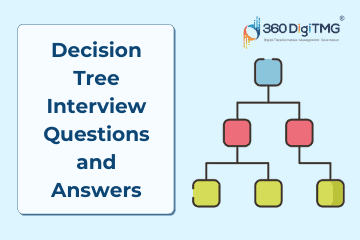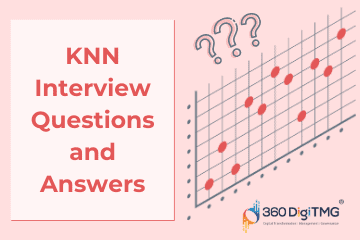Home / Blog / Interview Questions / Decision Tree Interview Q&A : Key Concepts in 2023

# Decision Tree Interview Q&A : Key Concepts in 2023

• February 04, 2023
• 15876
• 47### Meet the Author : Mr. Bharani Kumar

Bharani Kumar Depuru is a well known IT personality from Hyderabad. He is the Founder and Director of Innodatatics Pvt Ltd and 360DigiTMG. Bharani Kumar is an IIT and ISB alumni with more than 17 years of experience, he held prominent positions in the IT elites like HSBC, ITC Infotech, Infosys, and Deloitte. He is a prevalent IT consultant specializing in Industrial Revolution 4.0 implementation, Data Analytics practice setup, Artificial Intelligence, Big Data Analytics, Industrial IoT, Business Intelligence and Business Management. Bharani Kumar is also the chief trainer at 360DigiTMG with more than Ten years of experience and has been making the IT transition journey easy for his students. 360DigiTMG is at the forefront of delivering quality education, thereby bridging the gap between academia and industry.

• ### Decision tree algorithm belongs to the__________family.

• a) Supervised
• b) Unsupervised

• a) True
• b) False

• ### ______ is to create a training model that can be used to predict the class or value of the target variable by learning simple decision rules.

• a) Random Forest
• b) Decision Tree
• c) Both a and b
• d) None

• ### Identify the type of a decision tree________.

• a) Categorical
• b) Continuous
• c) Both a and b
• d) None

Answer - c) Both a and b

• a) 2
• b) 3
• c) 4
• d) Only 1

• a) Yes
• b) No

• a) Yes
• b) No

• ### ______is used for cutting or trimming the tree in Decision trees.

• a) Pruning
• b) Stemming

• ### Does the decision of making strategic splits heavily affects a tree’s accuracy?

• a) No
• b) Yes
• c) Maybe
• d) Don’t know

• ### ______ measure of the randomness in the information being processed in the Decision Tree.

• a) Entropy
• b) Information Gain

• ### ______is a statistical property that measures how well a given attribute separates the training examples according to their target classification.

• a) Entropy
• b) Information Gain

• ### _________computes the difference between entropy before the split and average entropy after the split of the dataset based on given attribute values.

• a) Information gain
• b) Gini ratio
• c) Pruning

• ### Information gain is biased towards choosing attributes with a large number of values as ______.

• a) Branch nodes
• b) Root nodes
• c) Leaf nodes

• a) Yes
• b) No

• ### ________is an algorithm used for continuous target variables that are used for regression problems in Decision Tree.

• a) Reduction in Variance
• b) Collinearity
• c) Correlation

Answer - a) Reduction in Variance

• ### Decision tree is a ___________.

• a) Non-linear ML technique.
• b) Non-Parametric technique.
• c) Supervised Learning technique.
• d) All of the above.

Answer - d) All of the above

• ### Choose the correct statement from below –

• a) A decision tree is a graphical representation of all the possible solutions to a decision based on certain conditions.
• b) Decision Trees usually mimic human thinking ability while making a decision, so it is easy to understand.
• c) A decision tree model consists of a set of rules for dividing a large heterogeneous population into smaller, more homogenous (mutually exclusive) classes.
• d) All of the above.

Answer - d) All of the above

• ### Decision tree is also referred to as__________________ algorithm.

• a) Recursive partitioning.
• b) Non-Recursive partitioning.
• c) Variable partitioning.
• d) None of the above.

• ### Decision tree is used for __________________.

• a) Only Regression.
• b) Only Classification.
• c) Both (a) and (b).
• d) None of the above.

Answer - c) Both (a) and (b)

• ### In the Decision tree, one rule is applied after another, resulting in a hierarchy of segments within segments. The hierarchy is called a __________, and each segment is called a ____________.

• a) Node, Tree.
• b) Tree, Node.
• c) Branch, Node.
• d) None of the above.

• ### Choose the correct sequence of typical decision tree structure –

(I) Take the entire data set as input
(II) Divide the input data into two part
(III) Reapply the split to every part recursively
(IV) Stop when meeting desired criteria
(V) Cut the tree when we went too far while doing splits(pruning)
• a) (I), (II),(V),(IV),(III).
• b) (V),(I),(III),(II),(IV).
• c) (I),(III),(II),(V),(IV).
• d) (I),(II),(III),(IV),(V).

• ### ___________ denotes the entire population or sample and it further divides into two or more homogeneous sets.

• a) Leaf node.
• b) Terminal node.
• c) Root node.
• d) None of the above.

• ### ___________ Node are those that do not split into parts.

• a) Root.
• b) Leaf / terminal.
• c) Branch.
• d) None of the above.

Answer - b) Leaf / terminal

• ### The Process of removing sub-nodes from a decision node is called ___________.

• a) Splitting.
• b) Breaking.
• c) Pruning.
• d) None of the above.

• ### Decision tree classifier is achieved by __________ splitting criteria.

• a) Entropy.
• b) Information Gain.
• c) Gini Index.
• d) All of the above.

Answer - d) All of the above

• ### Decision tree regressor is achieved by __________ splitting criteria

• a) Loss in the mean squared error.
• b) Entropy.
• c) Information Gain.
• d) None of the above.

Answer - a) Loss in the mean squared error

• ### ___________ is a measure of uncertainty of a random variable.

• a) Information gain.
• b) Entropy.
• c) Gini Index.
• d) None of the above.

• ### Information gain is required to decide ___________.

• a) Which feature to split on at every step in building the tree.
• b) Possible combinations of variables.
• c) No. of possible branches.
• d) All of the above.

Answer - a) Which feature to split on at every step in building the tree

• ### ____________ is a measurement of likelihood of an incorrect classification of a new instance for a random variable, if the new instance is randomly classified as per the distribution of class labels from the data set.

• a) Gini impurity.
• b) Entropy.
• c) Information gain.
• d) None of the above.

• ### In Decision tree pruning methods include

• a) No pruning.
• b) Reduced error pruning.
• c) Bagging.
• d) All of the above.

Answer - d) All of the above

• ### What is the regularization parameter in Decision tree?

• a. Regularization
• b. max_depth
• c. min_length
• d. None of the above

• ### Which argument we need to pass in decision tree to make the algorithm boosting algorithm?

• a.Entropy
• b. Trial
• c. Gini
• d. None of the above

• ### Which nodes have the maximum Gini impurity in a decision tree?

• a. 0.6
• b. 0.1
• c. 0.5
• d. none of the above

• a. True
• b.False

• ### Which algorithm is most prune to overfitting?

• b. Neural Analysis
• c. Decision Tree
• d. None of the above

• ### In which pruning method we allow our tree to grow till last including all the observations and variables?

• a. post prunning
• b. pre prunning
• c. both a & b
• d. None of the above

• ### According the value of ------ we split the node and split the decision tree?

• a. Gini Index
• b. Entropy
• c. Information Gain
• d. None of the above

• ### Entropy is similar to a.estimated mean b.estimated information event

• a. both a and b
• b. only b
• c. only a
• d. neither a and b

• ### Gene index is almost similar to a. estimated mean b. estimated information event

• a. both a and b
• b. only b
• c. only a
• d. neither a and b

• ### Pre-pruning the decision tree may results in

• a. Overfitting
• b. Underfitting

• ### Statement : Missing data can be handled by the DT. reason : classification is done by the yes or no condition.

• a. statment is True, Reason gives the correct explanation of statement
• b. statement is false, Reason is correct
• c. statement is false, reason is incorrect
• d. statement is true, reason is false explaination of statement

Answer - a. statment is True, Reason gives the correct explanation of statement

• ### Leaf node in a decision tree will have entropy value

• a. equal to zero
• b. equal to 1
• c. none

Answer - a. equal to zero

• a. 1
• b. 0
• c. None

• ### Which measure select the best attribute to split the records?

• a.export_text
• b. Attribute Selection Measures(ASM)
• c. tree.plot_tree(clf)
• d. None of these

Answer - b. Attribute Selection Measures(ASM)

• ### To plot the tree we use

• a.export_text
• b. Attribute Selection Measures(ASM)
• c. tree.plot_tree(clf)
• d. None of these